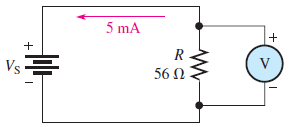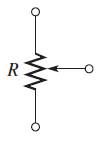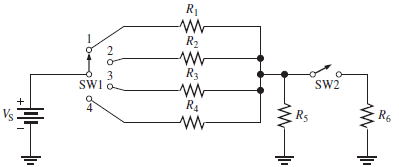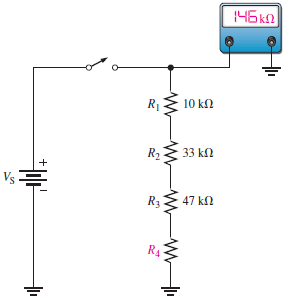# SSC JE Electrical Important MCQ Part 18

SSC JE Electrical Important MCQ PDF Part 18

1.How much voltage will be measured across the resistor in FigureA.280 V
B.280 mV
C.400 V
D.56 mV

Answer  : B (V= IR =5 mA×56 Ohms=5×10⁻³×56 = 280 mV)

2.An alternating voltage is represented by the expression v=35 sin(314.2 t ) volt.The period of the waveform is

A.50 ms
B.20 ms
C.50 s
D.20 s

(v=35 sin(314.2t) volt and comparing this to the standard v=Vm sin (2πft) volt we get 2πft=314.2
So f=314.2/2π=50 Hz
T=1/f=1/50 second=20 ms)

3.A sinusoidal alternating voltage has an average value of 3.5 V and a period of 6.67 ms.The standard expression for voltage will be

A.v=3.5 sin(314.2t)
B.v=5.5 sin(300πt)
C.v=5.5 sin(94.2t)
D.v=3.5 sin(30πt)

(Vav=3.5 V,T=6.67×10⁻³ s
Vav=0.637 Vm
So Vm=Vav/0.637=3.5/0.367=5.5 V
f=1/T=1/6.67×10⁻³ Hz=150 Hz
The standard expression is of the form v=Vm sin (2πft) volt
v=5.5 sin(2π×150×t)
v=5.5 sin(300πt) volts )

4.A non-sinusoidal waveform has a peak factor of 2.5, and an r.m.s. value of 240 V,The maximum value of voltage is

A.1.414 V
B.200 V
C.600 V
D.240 V

Answer  : C (Peak factor=maximum value/r.m.s. value So maximum value Vm= Peak factor×r.m.s.=2.5×240=600 V)

5.A battery of emf 6 V has an internal resistance of 0.15 ohms .What is its terminal p.d. when delivering a current of 0.5 A

A.5.925 V
B.6.000 V
C.4.000 V
D.7.075 V

Answer  : A (E=6 V,r=0.15 ohms,V=E-Ir=6-(0.5*0.15)=6-0.075=5.925 V)

6.A current of 200 mA flows through a resistance of 750 ohms for a time of 5 minutes,What is the energy dissipated

A.90 kJ
B.9 kJ
C.800 J
D.80 kJ

Answer  : B (W=I²Rt=0.2×750×300=9000 J or 9 kJ)

7.A resistor of 680 ohms,when connected in a circuit, dissipates a power of 85 mW. Then current flowing through it

A.7.6 A
B.7.6 mA
C.11.18 mA
D.11.18 A

Answer  : C (P=I²R So I=√P/R=√85×10⁻³/680=√1.25×10⁻⁴=11.18 mA)

8.Symbol shown in figure ofA.Resistor
B.Potentiometer
C.Rheostat
D.SPDT switch

Answer  : B (A potentiometer is a three-terminal resistor with a sliding or rotating contact that forms an adjustable voltage divider. If only two terminals are used, one end and the wiper, it acts as a variable resistor or rheostat)

9.Through which resistor in Figure is there always current, regardless of the position of the switchesA.R₁
B.R₃
C.R₅
D.R₆

10.The value of R₄ in the circuit of Figure isA.56 Ω
B.56 kΩ
C.90 kΩ
D.90 Ω

(From the ohmmeter reading,R total=146 kΩ
RT=R₄+R₁ + R₂ + R₃)
R₄ = RT – (R₁ + R₂ + R₃) = 146 kΩ – (10 kΩ + 33 kΩ + 47 kΩ) = 56 kΩ)

11.In three-phase transformer, maximum voltage regulation occurs at____________

A.Unity power factor
B.Rated KVA of transformer
D.Lagging power factor

12.In three phase star connection, line current is equal to _____________

A.Phase current
B.Phase current/√3
C.Phase voltage
D.Phase voltage/√3

13.The resistance of shunt field 250 V DC shunt generator is 200 ohms. What will be the field current

A.0 .8 Amps
B.4.5 Amps
C.1.25 Amps
D.5 Amps

Answer  : C (If=250/200=1.25 Amps )

14.In DC generator, armature is laminated to reduce _______

A.Field flux
B.Copper loss
C.Eddy current loss
D.Differentially compound generator

15.The 3 phase transformer used to transmit the voltage is connected in ——–

A.Star – Star
B.Delta – Delta
C.Delta – Star
D.Star – Delta

Answer  : C (Delta-Star transformers are common in commercial, industrial, and high-density residential locations, to supply three-phase distribution systems)

16.__________ relay is connected in the pipe connecting main tank of transformer and conservator.

A.Overcurrent
B.Distance
C.Buchholz
D.Directional

17.What is the full form of XLPE Cable

18.Energy meter is an example for which type of instrument

A.Pneumatic type
B.Recording type
C.Indicating type
D.Integrating type

Answer  : D (The integrating instrument measures the total energy supplied by the circuit in a given interval of time)

19.__________ is used for high switching frequency application.

A.TRIAC
B.MOSFET
C.SCR
D.Diode